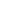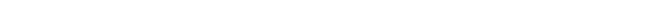The equivalence of magnetic and kinetic energy - “From Paradox to Paradigm”## The equivalence of magnetic and kinetic energy

When you are interested in physics you must read “Unbelievable“!

An electric charge placed in vacuum produces an electrostatic field that surrounds the charge. When an observer moves relatively to a charge, the observed electrostatic field changes in time. The observer moving relatively to the charge will, apart from measuring an altering electrostatic field, measure a magnetic field due to the relative movement to the charge. The presence of a magnetic field indic

ates magnetic energy.

For an observer, moving relatively to a mass, the relative speed of the mass presents kinetic energy. Kinetic energy exists only if there is relative movement.

Kinetic and magnetic energy are comparable in the way that both forms of energy exist only when there is relative motion of mass respectively electric charge to an observer.

An electron is a mass and charge that moves relatively to an observer and therefore presents both kinetic and magnetic energy. We will consider only non-relativistic velocities, because relativistic conditions unnecessarily complicate the situation without adding any additional insight.

The questions I want to be answered are

• How much energy does the magnetic field of a moving charge present?
• What is the relation between the magnetic and kinetic energy of a charged mass?Automated page speed optimizations for fast site performance